We present efficient algorithms for using selected configuration interaction (sCI) trial wave functions in phaseless auxiliary field quantum Monte Carlo (ph-AFQMC). These advances, geared toward optimizing computational performance for longer configuration interaction expansions, allow us to use up to a million configurations in the trial state for ph-AFQMC. In one example, we found the cost of ph-AFQMC per sample to increase only by a factor of about 3 for a calculation with 104 configurations compared to that with a single one, demonstrating the tiny computational overhead due to a longer expansion. This favorable scaling allows us to study the systematic convergence of the phaseless bias in auxiliary field quantum Monte Carlo calculations with an increasing number of configurations and provides a means to gauge the accuracy of ph-AFQMC with other trial states. We also show how the scalability issues of sCI trial states for large system sizes could be mitigated by restricting them to a moderately sized orbital active space and leveraging the near-cancellation of out of active space phaseless errors.

Quantum Monte Carlo (QMC) is a powerful tool in our arsenal to tackle the quantum many-body problem.1–7 Among various QMC approaches, phaseless auxiliary-field quantum Monte Carlo (ph-AFQMC)8 has emerged as an accurate and efficient method. While originally from the condensed matter community [therein usually referred to as constrained-path auxiliary field quantum Monte Carlo (AFQMC)],9–11 ph-AFQMC has gained popularity in chemistry in recent years.12–24 The accuracy and scalability of ph-AFQMC are determined in large part by the choice of the trial wave function. The use of a trial wave function becomes necessary for retaining statistical efficiency (in sample complexity) to control the fermionic phase (or sign) problem.25,26 The constraint imposed to control the phase problem is called the phaseless approximation.8 When the trial wave function approaches the exact ground state, the corresponding ph-AFQMC energy tends to the ground state energy. The commonly used trial wave function for ph-AFQMC is the broken-symmetry Hartree–Fock (HF) wave function. It scales as O(N3) to obtain the trial wave function and O(N5) to perform the ph-AFQMC calculation with the trial to obtain energy for a fixed statistical error, where N is the system size. Beyond HF, one may try to use a single determinant trial using approximate Bruckner orbitals,23 which essentially keeps the same computational scaling for ph-AFQMC. While single determinant trial wave functions are attractive due to their scalability, their accuracy can be limited and questionable in many examples.13,21,27

On the other hand, there has been a flurry of developments in selected configuration interaction (sCI) methods in the last few years.28–31 Despite their steep (formally exponential) scaling with system size, sCI methods have increasingly been employed to study moderately sized systems and perform large active space correlated calculations. These methods have the capability of generating systematically more accurate approximations to the state of interest by increasing the number of configurations judiciously. This attractive feature makes sCI wave functions natural candidates for trial states in projector QMC methods. Based on significant advances in real-space QMC algorithms with such trial states,32,33 several papers have already reported calculations with long sCI expansions in diffusion Monte Carlo.34–37 There has also been some recent progress for using them in ph-AFQMC,21,24,38 but the commonly used algorithm based on the Sheman–Morrison–Woodbury identity has an inherent computational bottleneck with the local energy evaluation scaling as O(NcN4), where Nc is the number of determinants. This steeper scaling compared to real-space methods is due to the Gaussian orbital representation of the electron repulsion interaction. Furthermore, the force bias evaluation scales as O(NcN2 + N3), which can be expensive since it is performed once every time step. Due to these, it has been challenging to scale up the ph-AFQMC calculations beyond 100–1000 configurations.

In this work, we propose to use one of the sCI methods, heat-bath CI (HCI),30,39,40 to generate a large trial with up to 106 configurations. We then combine several algorithmic advances made by two of us27,41 using the generalized Wick’s theorem and make further improvements to accelerate ph-AFQMC calculations with a large trial. In particular, in our new algorithm, the local energy evaluation scales as O(NcN + N4), and the force bias evaluation scales as O(Nc + N3). To alleviate the scaling issues of sCI wave functions, we study the efficacy of active space trial states in calculating energy differences and properties. The active space size is another variable to converge the phaseless error systematically in larger systems while keeping the calculation cost manageable. We use the new algorithm for ph-AFQMC with HCI trials to investigate the behavior of the phaseless bias as a function of the number of configurations in the trial for several challenging systems.

This paper is organized as follows: We first review the ph-AFQMC algorithm (Sec. II); we then discuss how one can drastically speed up the evaluation of force bias and local energy of sCI trials using the generalized Wick’s theorem (Sec. III); we show how phaseless errors in the ground state energy and dipole moments change as a function of the number of determinants in hydrogen chains, transition metal oxides, and a few small molecules (Sec. IV); and we finally conclude (Sec. V).

We briefly summarize the procedure for phaseless AFQMC here and refer the reader to Ref. 16 for more details. Consider the ab initio Hamiltonian given by

$Ĥ=∑ijhijâi†âj+12∑γ∑ijLijγâi†âj2,$
(1)

where hij are one-electron integrals and $Lijγ$ are Cholesky decomposed two-electron integrals in an orthonormal orbital basis. We will use letters N, M, and X to denote the number of electrons, the number of orbitals, and the number of Cholesky vectors, respectively. We note that in chemical systems, empirically, XO(M) with a proportionality constant usually smaller than 10. For density fitting (not employed here), we expect X ∼ 4 − 5M.42 AFQMC uses the exponential form of the projector to converge to the ground state of the Hamiltonian as

$e−τĤψI→τ→∞Ψ0,$
(2)

where τ is the imaginary time, $Ψ0$ is the ground state, and $ψI$ is an initial state such that $ψI|ψ0≠0$. Using the Hubbard–Stratonovic transform,43,44 the short-time exponential projector can be written as

$e−ΔτĤ=∫dxp(x)B̂(x),$
(3)

where x is the vector of auxiliary fields (one scalar field per Cholesky component), p(x) is the standard normal Gaussian distribution of the auxiliary fields, and $B̂(x)$ is a complex propagator given by the exponential of a one-body operator. Due to Thouless’ theorem,45 $B̂(x)$ acts on a Slater determinant $ϕ$ as

$B̂(x)ϕ=ϕ(x),$
(4)

where $ϕ(x)$ is another Slater determinant obtained by a linear transformation of the orbitals in $ϕ$. By sampling the auxiliary fields in Eq. (3) and applying the short time propagator sufficiently many times, we obtain a stochastic representation of the ground state wave function in the long time limit as

$Ψ0∝∑iwiϕiψT|ϕi,$
(5)

where wi are weights, $ϕi$ are Slater determinants with complex orbitals sampled during propagation, and $ψT$ is the trial state used for importance sampling. More accurate trial states lead to less noisy weights.

We use the hybrid approximation8 in this paper, which avoids the expensive calculation of local energy at each propagation step. Importance sampling in AFQMC involves shifting the sampled auxiliary fields by the force bias given as

$x̄γ=−ΔτψT∑ijLijγâi†âjϕψT|ϕ,$
(6)

where $ϕ$ is the walker. The force bias can be thought of as a dynamic correction to the mean-field contour shift46 that vanishes as Δτ → 0. Force bias is not enough, by itself, to control the large fluctuations stemming from the phase problem. The phaseless constraint8 can be used to overcome the phase problem at the expense of a systematic bias in the sampled wave function. The size of this phaseless bias is dictated by the accuracy of the trial state.

After an equilibration time, the energy of the sampled wave function can be measured as

$E≈∑iwiEL(ϕi)∑iwi,$
(7)

where

$EL(ϕi)=ψTĤϕiψT|ϕi$
(8)

is the local energy of the walker $ϕi$. We note that this estimator has a zero-variance property, i.e., in the limit that the trial state is the exact, ground state the variance of the estimator vanishes. In practice, this means that more accurate trial states lead to less noisy local energy values.

It is evident that more accurate trial states lead to less noisy simulations and smaller phaseless biases. However, in general, the use of more sophisticated trial states comes at the expense of greater computational cost. In Sec. III, we present efficient algorithms to calculate force bias and local energy of sCI trial states.

A selected CI wave function is given by

$ψT=∑nNccnψn=∑nNccn∏μknâtnμ†âpnμψ0,$
(9)

where $ψn$ are configurations obtained by particle–hole excitations from the reference configuration $ψ0$, Nc is the number of configurations in the expansion, cn are real expansion coefficients, and kn are the excitation ranks. We will use the indices pμ and tμ to denote occupied and virtual orbitals, respectively, whereas indices i, j, … will be used for general orbitals. The orthonormal orbital basis set used in the expansion can be chosen to be natural orbitals obtained from an HCI calculation or can be optimized using a self-consistent procedure. In the following, we use the same basis set to express the Hamiltonian in Eq. (1) and, in general, all second-quantized operators refer to this basis. In our prior work, we have proposed efficient algorithms for using selected CI states in variational Monte Carlo41 and free projection AFQMC.27 These focused on the calculation of local energy using the generalized Wick’s theorem. Here, we discuss an algorithm for calculating the force bias required in ph-AFQMC and briefly summarize the calculation of local energy.

The calculation of the force bias for importance sampling is one of the computationally intensive parts of propagation in ph-AFMQC. By substituting the sCI trial state into the force bias expression in Eq. (6), we obtain

$x̄γ=−Δτ∑n∑ijcnLijγψnâi†âjϕ∑ncnψn|ϕ.$
(10)

The conventional way of evaluating the force bias proceeds by separately calculating the contribution of each configuration in the CI expansion using the Sherman–Morrison–Woodbury identity, resulting in a cost scaling of O(NcNM + XNM).38 Here, we present an algorithm with cost scaling as O(Nc + XM2) by essentially separating the sum over CI excitations from that over Cholesky indices in Eq. (10).

We define the Green’s function matrix for the reference configuration $ψ0$ and the walker configuration $ϕ$ as47,48

$Gji=ψ0âi†âjϕψ0|ϕ=ϕ(ψ0†ϕ)−1ψ0†ij,$
(11)

where ψ0 and ϕ are the orbital coefficient matrices of the corresponding configurations and superscripts and subscripts denote row and column indices, respectively. Note that since we work in the orbital basis of the CI expansion, ψ0 has a particularly simple form with all its columns being unit vectors. Thus, the cost of calculating Green’s function in this basis scales as O(N2M). For convenience, we also define the related quantity as

$Gji=−ψ0âjâi†ϕψ0|ϕ=Gji−δji.$
(12)

According to the generalized Wick’s theorem,48 we have

$ψ0∏μkâpμ†âtμϕψ0|ϕ=detGtμpμ,$
(13)

where the sets of indices $pμ$ and $tμ$ denote the k × k slice of the G matrix, with k being the rank of the excitation. This expression is obtained by taking pairwise contractions of the operators in the string of excitations according to the generalized Wick’s theorem with the determinant structure arising due to fermionic permutation parity factors. Therefore, the denominator in Eq. (10) can be expressed as

$ψT|ϕψ0|ϕ=∑ncndetGtnμpnμ.$
(14)

The computational cost scaling of this overlap ratio is, thus, O(Nc) once Green’s function has been calculated. Since Wick’s theorem applies naturally to overlap ratios, we will find it convenient to express matrix elements as ratios with the reference overlap $ψ0|ϕ$.

To evaluate the numerator of Eq. (10), consider the matrix element ratio given by

$ψnâi†âjϕψ0|ϕ=ψ0∏μkâpμ†âtμâi†âjϕψ0|ϕ,$
(15)

where we have dropped the configuration index subscript (n) on the excitations for clarity. This ratio can also be evaluated using the generalized Wick’s theorem, and by choosing the appropriate order of operations, one can achieve a significant reduction in the cost of calculating the numerator. To this end, we note that the pairwise contractions in the string of excitation operators can be divided into two groups: (1) those without any contractions between CI excitations and $âi†âj$, (2) those containing contractions between two of the CI operators and $âi†âj$. Algebraically, the two terms are given as

$ψ0∏μkapμ†atμâi†âjϕψ0|ϕ=detGjiGtμiGjpμGtμpμ=GjidetGtμpμ+∑ν,λk(−1)1+ν+λGjpνGtλidetGtμ\tλpμ\pν,$
(16)

where on the first line, the matrix of size (k + 1) × (k + 1) is written in a block form, and the notation $pμ\pν$ indicates the set of indices $pμ$ excluding pν. Using this grouping of terms, we get for the numerator in Eq. (10),

$∑n∑ijcnLijγψnâi†âjϕψ0|ϕ=∑ncndetGtμnpμn∑ijLijγGji+∑ptIpt∑ijLijγGjpGti,$
(17)

where

$Ipt=∑ncn∑ν,λknδpnν,pδtnλ,t(−1)1+ν+λdetGtnμ\tnλpnμ\pnν$
(18)

is an intermediate formed by summing over the CI configurations. The two terms in Eq. (17) are shown as tensor contraction diagrams in Table I for the case of doubly excited CI configurations. Note that a selected CI state does not necessarily include all excitations of a given rank; however, for a tensor representation, we consider all doubly excited configurations in the table. In practice, the sum over CI excitations in Table I is only performed over the configurations in the selected CI state. The first term has a cost scaling of O(Nc + XNM), while the second one using the intermediate has a scaling of O(Nc + XM2). If the selected CI state is restricted to an active space of size A, the overall cost can be reduced to O(Nc + XNM + XAM).

TABLE I.

Summary of computational cost scaling of force bias and two-body local energy calculations (given the reference Green’s function matrix G). Sherman–Morrison–Woodbury (SMW) refers to overall cost scaling of the algorithm that makes use of the Sherman–Morrison–Woodbury formula as described in Ref. 38. The last columns shows tensor contraction diagrams for all distinct types of pairwise Wick’s contractions using all doubly excited configurations $∑cptquât†âpâu†âqψ0$ as an example. Combining all possible contractions of a given type along with the fermionic parity signs (not shown here) leads to the determinant expressions in Eqs. (17) and (20). The same types of terms arise for higher than doubly excited configurations, and the adjacent column shows the optimal cost scaling for calculating each type for a general CI trial with Nc configurations.

This worka
QuantitySMWScalingTensor diagrams for doubly excited configurations
Force bias NM(Nc + XNc + XNM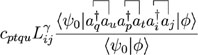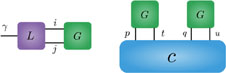Local energy NcXN2M Nc + XM2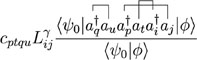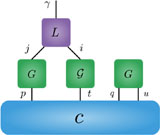Nc + XNM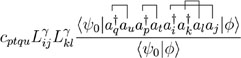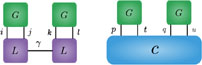Nc + XN2M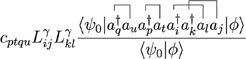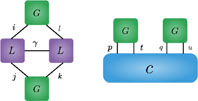Nc + XM2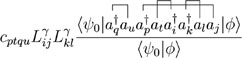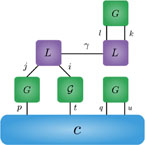Nc + XNM2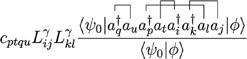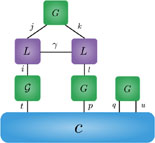X(Nc + NM2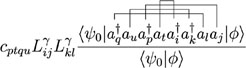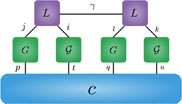This worka
QuantitySMWScalingTensor diagrams for doubly excited configurations
Force bias NM(Nc + XNc + XNMLocal energy NcXN2M Nc + XM2Nc + XNMNc + XN2MNc + XM2Nc + XNM2X(Nc + NM2a

Nc: number of configurations; N: Number of electrons; M: Number of orbitals; X: Number of Cholesky vectors; c: CI coefficients; L: Cholesky vectors; G and $G$: Green’s functions; i, j, k, l: Hamiltonian indices; and p, q, t, u: CI excitation indices.

The calculation of local energy was discussed in detail in Ref. 27. Here, we give a brief summary of the algorithm. Consider the two-body part of the local energy [see Eq. (8)] expressed as

$EL2ϕ=∑n∑γijklcnLijγLklγψnâi†âk†âlâjϕψ0|ϕ∑ncnψn|ϕψ0|ϕ.$
(19)

The calculation of the overlap ratios in the denominator is described in Sec. III A. The matrix element in the numerator for the nth configuration is given by using Wick’s theorem as

$ψ0∏μkâpμ†âtμâi†âk†âlâjϕψ0|ϕ=detGj,li,kGtμi,kGj,lpμGtμpμ,$
(20)

where we have again dropped the configuration index subscript. Similar to the force bias calculation, the terms in this determinant can be split into two groups based on the kinds of pairwise contractions: those that do not involve cross-contractions between CI and Hamiltonian excitation operators and those that involve at least one such cross-contraction. The second group containing cross-contractions can be further split into two groups depending on whether one or both of the Hamiltonian excitations are cross-contracted with CI excitations. The resulting terms are shown as a tensor diagram in Table I. Again, we assume all double excited CI configurations only for representational convenience. Different orders of performing the tensor contractions lead to different cost scalings, and using the one described in Ref. 27 leads to a scaling of O(NcX + XNM2). For a selected CI state restricted to an active space of size A, this cost is reduced to O(NcX + XNAM + XN2M).

In this section, we present the results of our ph-AFQMC/HCI calculations and analyze the convergence of the phaseless error for several systems. In particular, we consider the utility of active space trial states for obtaining accurate energy differences and dipole moments. We used PySCF49 to obtain molecular integrals and to perform all quantum chemistry wave function calculations. The SHCI code Dice was used to obtain the trial states. We do not converge the HCI variational calculations close to near-exact accuracies but only generate relatively crude expansions at a modest computational cost. The code used to perform ph-AFQMC calculations is available in a public repository.50 Input and output files for all calculations can also be accessed from a public repository.51 Sources of systematic errors in ph-AFQMC calculations, besides the phaseless bias, include Trotter error, population control bias, and errors in the Cholesky decomposition of the electron repulsion integrals, matrix exponential Taylor series truncation errors, and bias due to filtering of rare large fluctuations. We used a conservative time step of 0.005 a.u. in all ph-AFQMC calculations. For population control, we used the reconfiguration procedure described in Ref. 52. Cholesky decompositions were calculated up to a threshold error of 10−5. Matrix exponentials were calculated by keeping terms up to the sixth power in the Taylor expansion. We filtered large fluctuations by capping weights and local energies.16 Although it is difficult to get an accurate estimate of the systematic errors due to all these factors in general, they can be controlled systematically. We estimate these errors to be much smaller than the statistical errors in all the results presented here.

Hydrogen chain systems are convenient for benchmarking due to their simplicity and the availability of accurate density matrix renormalization group (DMRG) ground state energies.53 First, we consider a chain of 50 equidistant hydrogen atoms with an interatomic distance d = 1.6 Bohr in the minimal STO-6G basis. We performed ph-AFQMC/HCI calculations on this system using progressively larger HCI expansions. These trial states were constructed as truncations of the HCI state obtained with ϵ1 = 10−4 in the canonical RHF basis. Figure 1 shows the convergence of the phaseless error (calculated as a difference to the DMRG energy reported in reference) with the number of configurations in the trial state. We observe a nearly monotonic decrease in the phaseless error, and the error is less than 2 mH for the trial with 106 configurations. The figure also shows the scaling of the cost of the ph-AFQMC calculation against the number of configurations with respect to the cost of a single configuration calculation. Remarkably, the bare cost factor for up to 104 configurations is less than 10 and increases roughly linearly with the number of configurations thereafter. This observation is consistent with the scaling relations discussed in Sec. III, with the O(NcX) factor in the cost scaling of local energy evaluation dominating for large HCI expansions. We also show an error adjusted cost factor to estimate the cost for obtaining a fixed stochastic error. Since more accurate trial states lead to a reduced stochastic noise, this adjusted cost factor is less than one for Nc ≤ 104, demonstrating the efficacy of these trial states. We found that changing the value of ϵ1 or slightly changing the selection criterion for choosing the configurations only makes a difference in ph-AFQMC energies for the smaller number of configurations, leading to similar convergence behaviors for longer expansions.

FIG. 1.

Convergence of the ph-AFQMC/HCI phaseless energy error and scaling of the cost of computation with the increasing number of configurations for H50 using the STO-6G basis at d = 1.6 Bohr. The phaseless error is calculated with respect to the near-exact DMRG energy. The bare cost factor is evaluated as the ratio of computational costs with respect to a single configuration calculation using the same number of samples. The adjusted cost factor is calculated as the bare cost factor $×σNc/σ12$ to estimate the cost to obtain a fixed stochastic error.

FIG. 1.

Convergence of the ph-AFQMC/HCI phaseless energy error and scaling of the cost of computation with the increasing number of configurations for H50 using the STO-6G basis at d = 1.6 Bohr. The phaseless error is calculated with respect to the near-exact DMRG energy. The bare cost factor is evaluated as the ratio of computational costs with respect to a single configuration calculation using the same number of samples. The adjusted cost factor is calculated as the bare cost factor $×σNc/σ12$ to estimate the cost to obtain a fixed stochastic error.

Close modal

We also studied the H10 chain in the cc-pVDZ basis at different bond lengths to gauge the performance of active space trial states. We first performed a (10e, 10o) complete active space self-consistent field (CASSCF) calculation and generated trial states by truncating the active space wave function. Figure 2 shows the convergence of the phaseless error with the size of the HCI trial. For all three bond lengths, we see a systematic convergence to an almost vanishing error with the active space trial. The shortest bond length near equilibrium converges rapidly, while the stretched bond length requires almost the full active space wave function to reach convergence. The out of active space phaseless error, arising due to not correlating any orbitals outside the active space in the trial state, is tiny for all three bond lengths in this case. We also show ph-AFQMC/UHF phaseless errors in the figure for comparison. ph-AFQMC/UHF is known to be very accurate for hydrogen chains except at stretched geometries. At stretched geometries, a CI expansion based on an unrestricted HF reference has been employed in the past as a trial state53 and could be a handy tool in other problems as well. The algorithms described in this work can be straightforwardly extended to work with UHF-based CI expansions.

FIG. 2.

ph-AFQMC/HCI phaseless errors for H10 using the cc-pVDZ basis set at different bond lengths. The trial states with different numbers of configurations are constructed by truncating a (10e, 10o) CASSCF wave function in each case. The hollow symbols show ph-AFQMC/UHF phaseless errors. (They are almost zero for d = 1.6 Bohr and d = 2.4 Bohr.)

FIG. 2.

ph-AFQMC/HCI phaseless errors for H10 using the cc-pVDZ basis set at different bond lengths. The trial states with different numbers of configurations are constructed by truncating a (10e, 10o) CASSCF wave function in each case. The hollow symbols show ph-AFQMC/UHF phaseless errors. (They are almost zero for d = 1.6 Bohr and d = 2.4 Bohr.)

Close modal

Recent studies of transition metal oxide molecules reported benchmark ground state energies using various accurate many-body methods.21,54 Here, we use ph-AFQMC/HCI to study convergence of the phaseless errors for active space trials in TiO, CrO, MnO, and FeO. The Trails-Needs pseudopotential and the corresponding DZ basis sets55 were used at equilibrium geometries used in Ref. 21.

Figure 3 shows the convergence of phaseless errors with the number of configurations in the trial state at equilibrium geometries used in Ref. 21. We first performed CASSCF calculations with active spaces consisting of the metal 3d and 4d orbitals and oxygen 2p orbitals. The HCI trial states were then generated in a space including the CASSCF active orbitals and the core orbitals, including all electrons. ϵ1 = 10−5 was used for the HCI calculations. Interestingly, for FeO, CrO, and MnO, the phaseless error first increases before converging to a fixed value as the number of configurations in the trial is increased. This peculiar behavior highlights the fact that a lower energy trial state does not guarantee a smaller phaseless error. We note that the trends for smaller expansions are dependent on the particular schemes used for choosing the orbital spaces and for truncating the HCI states. However, results for a large number of configurations are largely independent of such ambiguity. For TiO, using more configurations makes little difference to the phaseless error. We also performed ph-AFQMC/UHF calculations for comparison and found the phaseless errors to be 2(1), 5.3(8), −10(1), and 30.6(9) mH for TiO, CrO, MnO, and FeO, respectively.

FIG. 3.

Convergence of ph-AFQMC/HCI phaseless energy errors for four transition metal oxide molecules using the Trail-Needs dz pseudopotential and basis set. SHCI reference energies were used as references for calculating the phaseless errors. The trial states were constructed by truncating active space HCI wave functions. Hollow symbols show ph-AFQMC/UHF errors.

FIG. 3.

Convergence of ph-AFQMC/HCI phaseless energy errors for four transition metal oxide molecules using the Trail-Needs dz pseudopotential and basis set. SHCI reference energies were used as references for calculating the phaseless errors. The trial states were constructed by truncating active space HCI wave functions. Hollow symbols show ph-AFQMC/UHF errors.

Close modal

The residual phaseless errors can be attributed solely to the out of HCI active space correlation. These errors can be systematically reduced by increasing the size of the active space, but vanishing errors this way is unlikely to be practical for larger systems. On the other hand, as noted in Sec. IV A, it is reasonable to expect near-cancellation of this out of active space error when energy differences at different geometries are calculated using the same active space. Similar trends have been observed in multireference perturbation theories. To gauge the efficacy of this heuristic, we calculated ph-AFQMC/HCI energies of CrO at three bond lengths using the procedure described above. Table II shows that the converged phaseless errors are almost identical at the three points. We note that it is crucial to converge the phaseless error of the active space trial, and different geometries may require trial states with different numbers of configurations to achieve convergence. The table also shows energies at infinite separation, showing that the error in the ph-AFQMC/HCI dissociation energy compared to SHCI is 1.8(9) mH. This evidence of small non-parallelity errors suggests that in large problems, scalability issues of HCI trial states can be mitigated using active spaces.

TABLE II.

CrO ground state energies and out of active space phaseless errors at different bond lengths. Bond lengths (d) are in Bohr, ph-AFQMC/HCI and SHCI energies are in H, and the phaseless errors are in mH. The last line shows energies at dissociation.

dph-AFQMC/HCISHCIPhaseless error
2.65 −102.4993(5) −102.5040(5) 4.6(7)
3.06 −102.5531(5) −102.558421  5.3(5)
3.59 −102.5269(7) −102.5325(5) 5.6(8)
∞ −102.3862(8) −102.393321  7.1(8)
dph-AFQMC/HCISHCIPhaseless error
2.65 −102.4993(5) −102.5040(5) 4.6(7)
3.06 −102.5531(5) −102.558421  5.3(5)
3.59 −102.5269(7) −102.5325(5) 5.6(8)
∞ −102.3862(8) −102.393321  7.1(8)

The mixed estimator of an observable $Ô$ in the ground state is given by

$⟨O⟩mixed=ψTÔΨ0ψT|Ψ0,$
(21)

where $Ψ0$ is the ground state. In ph-AFQMC, one has access to an approximate sampling of the ground state, making the calculation of this mixed estimator convenient,

$⟨O⟩mixed≈∑iwiOL(ϕi)∑iwi,$
(22)

where

$OL(ϕi)=ψTÔϕiψT|ϕi$
(23)

is the local observable value.

For observables $Ô$ that do not commute with the system Hamiltonian, the mixed estimator has a systematic bias due to the trial state used to measure the observable. This bias can be reduced by using the extrapolated estimator, often employed in diffusion Monte Carlo,56–58 given by

$⟨Ô⟩extrapolated=2ψTÔΨ0ψT|Ψ0−ψTÔψTψT|ψT,$
(24)

where the variational estimator of the trial state is used to correct the mixed estimator. The accuracy of this extrapolation depends critically on the quality of the trial state. Since ph-AFQMC calculations on molecular systems are typically performed using crude single determinant trials, extrapolation is not a viable option, and backpropagation is used to evaluate variational estimators of the sampled state instead.59 Here, we study the behavior of the mixed and extrapolated estimators of dipole moments of a few small molecules. The evaluation of the local dipole moment for an HCI trial state is similar to the force bias calculation outlined in Sec. III A since they both involve matrix elements of one-electron operators.

We first look at the exact solvable problem of NH3 in the 6-31G basis (see the supplementary material for geometry). We fully diagonalize this (10e, 14o) problem and calculate its ground state dipole moment. Mixed and extrapolated ph-AFQMC estimators were evaluated with trial states consisting of an increasing number of leading configurations from the ground state in the RHF canonical orbital basis. Figure 4 shows the convergence of the estimators with the trial state. In this case, we see a systematic convergence of the variational and mixed estimators to the exact value. The extrapolation works reasonably well with consistently smaller errors than the mixed estimator.

FIG. 4.

Convergence of different estimators for the dipole moment of NH3 using the 6-31G basis. Variational and mixed estimators are calculated using HCI and ph-AFQMC/HCI, respectively.

FIG. 4.

Convergence of different estimators for the dipole moment of NH3 using the 6-31G basis. Variational and mixed estimators are calculated using HCI and ph-AFQMC/HCI, respectively.

Close modal

Table III shows the calculated dipole moments for three molecules at equilibrium geometries in the aug-cc-pVQZ basis. The large basis set precludes exact evaluation, but CCSD(T) moments are known to be very accurate for the molecules considered here and agree well with the experimental values. We computed orbital relaxed coupled cluster and MP2 dipole moments by calculating energies at two different electric fields and evaluating the numerical derivative. For obtaining ph-AFQMC/HCI estimators, we first performed a full-valence CASSCF calculations. The trial states were then constructed using HCI to correlate all electrons in a space of 50 CASSCF orbitals. In all cases, both mixed and extrapolated estimators were nearly converged with the number of configurations in the active space (details can be found in the supplementary material). Consider the case of the challenging CO molecule, which has a small dipole moment, and RHF predicts the wrong direction polarity in this case. Furthermore, MP2 overestimates the dipole moment significantly. The convergence of the dipole moment estimators with the number of configurations in the trial state is shown in Fig. 5. For a small number of configurations (fewer than 100), the variational estimator is qualitatively incorrect similar to RHF. Adding more configurations flips the sign, and the variational estimator seems to eventually converge to the asymptotic value for the truncated space of 50 orbitals. The mixed estimator also exhibits a non-monotonic convergence with its value for a single determinant close to the experimental value because of a fortuitous cancellation of mixed estimator and phaseless biases. The extrapolated estimator for the small number of configurations in the trial is very poor due to the erroneous variational estimates. For more than 103 configurations, while the mixed estimator seems to be converging to a substantially biased dipole moment value, the extrapolation rectifies this bias reasonably well. The extrapolated estimators are in good agreement with the CCSD(T) and experimental values for all three molecules. Evidently, extrapolation effectively corrects the out of active space bias in the mixed estimator for CO and BF.

TABLE III.

Dipole moments (in a.u.) of three molecules using the aug-cc-pVQZ basis set. The reported ph-AFQMC/HCI values are converged with the number of configurations in the 50 orbital active space trial state.

ph-AFQMC/HCI
MoleculeMixedExtrapolatedRHFMP2CCSDCCSD(T)Experiment
CO 0.070(3) 0.044(6) −0.104 0.108 0.024 0.048 0.04860
BF 0.356(4) 0.321(8) 0.333 0.377 0.314 0.325 ⋯
H20.726(2) 0.734(4) 0.780 0.733 0.738 0.729 0.73061
ph-AFQMC/HCI
MoleculeMixedExtrapolatedRHFMP2CCSDCCSD(T)Experiment
CO 0.070(3) 0.044(6) −0.104 0.108 0.024 0.048 0.04860
BF 0.356(4) 0.321(8) 0.333 0.377 0.314 0.325 ⋯
H20.726(2) 0.734(4) 0.780 0.733 0.738 0.729 0.73061
FIG. 5.

Convergence of different estimators for the dipole moment of CO using the aug-cc-pVQZ basis. Variational and mixed estimators are calculated using HCI and ph-AFQMC/HCI, respectively. Trial states are obtained by truncating a (14e, 50o) active space HCI wave function.

FIG. 5.

Convergence of different estimators for the dipole moment of CO using the aug-cc-pVQZ basis. Variational and mixed estimators are calculated using HCI and ph-AFQMC/HCI, respectively. Trial states are obtained by truncating a (14e, 50o) active space HCI wave function.

Close modal

In this work, we presented efficient algorithms for using selected CI trial states in ph-AFQMC. We demonstrated their favorable scaling by showing that simulations with long sCI expansions incur little overhead compared to a single reference trial. In our analysis of the convergence of phaseless error in ground-state energies and dipole moments as a function of the number of configurations, we found it to be non-monotonic in some cases, highlighting the importance of using a sufficient number of determinants in these systems. The use of states restricted to moderately sized active spaces yielded excellent results for energy differences and dipole moments. Our numerical experiments suggest that this may be a practical way to tackle larger systems, where the generation and handling of sCI states in the full space are challenging. Finally, we showed that the extrapolated estimator for dipole moments is accurate if enough configurations are present in the trial state. If this behavior turns out to be true in general, extrapolated estimators could be a viable alternative to backpropagation for calculating ground state properties.

Our technique can be employed in different ways for studying challenging systems. It can be used to validate the use of simpler and less expensive trial states in ph-AFQMC for large calculations. ph-AFQMC/HCI can be adopted as an accurate solver in embedding schemes for describing the embedded cluster. It would be interesting to study the feasibility of the extrapolated estimator in larger systems and for calculating two-body properties. Finally, we note that this technique can be utilized in the quantum–classical hybrid AFQMC (QC-AFQMC) for prototypical and practical quantum computations.62

See the supplementary material for geometries and details of wave functions used.

A.M. and S.S. were supported by the National Science Foundation through Grant No. CHE-1800584. S.S. was also partly supported through the Sloan research fellowship. J.L. thanks David Reichman for support and encouragement. All calculations were performed on the Blanca and Summit clusters at CU Boulder.

The authors have no conflicts to disclose.

The data that support the findings of this study are available in a public repository in Ref. 51.

1.
M. H.
Kalos
,
D.
Levesque
, and
L.
Verlet
, “
Helium at zero temperature with hard-sphere and other forces
,”
Phys. Rev. A
9
,
2178
(
1974
).
2.
D.
Ceperley
,
G. V.
Chester
, and
M. H.
Kalos
, “
Monte Carlo simulation of a many-fermion study
,”
Phys. Rev. B
16
,
3081
(
1977
).
3.
D. M.
Ceperley
and
B. J.
Alder
, “
Ground state of the electron gas by a stochastic method
,”
Phys. Rev. Lett.
45
,
566
(
1980
).
4.
M. P.
Nightingale
and
C. J.
Umrigar
,
Quantum Monte Carlo Methods in Physics and Chemistry
(
,
1998
).
5.
W. M. C.
Foulkes
,
L.
Mitas
,
R. J.
Needs
, and
G.
Rajagopal
, “
Quantum Monte Carlo simulations of solids
,”
Rev. Mod. Phys.
73
,
33
(
2001
).
6.
G. H.
Booth
,
A.
Grüneis
,
G.
Kresse
, and
A.
Alavi
, “
Towards an exact description of electronic wavefunctions in real solids
,”
Nature
493
,
365
(
2013
).
7.
F.
Becca
and
S.
Sorella
,
Quantum Monte Carlo Approaches for Correlated Systems
(
Cambridge University Press
,
2017
).
8.
S.
Zhang
and
H.
Krakauer
, “
Quantum Monte Carlo method using phase-free random walks with Slater determinants
,”
Phys. Rev. Lett.
90
,
136401
(
2003
).
9.
S. B.
Fahy
and
D. R.
Hamann
, “
Positive-projection Monte Carlo simulation: A new variational approach to strongly interacting fermion systems
,”
Phys. Rev. Lett.
65
,
3437
(
1990
).
10.
S.
Zhang
,
J.
Carlson
, and
J. E.
Gubernatis
, “
Constrained path quantum Monte Carlo method for fermion ground states
,”
Phys. Rev. Lett.
74
,
3652
(
1995
).
11.
S.
Zhang
,
J.
Carlson
, and
J. E.
Gubernatis
, “
Constrained path Monte Carlo method for fermion ground states
,”
Phys. Rev. B
55
,
7464
(
1997
).
12.
W. A.
Al-Saidi
,
S.
Zhang
, and
H.
Krakauer
, “
Auxiliary-field quantum Monte Carlo calculations of molecular systems with a Gaussian basis
,”
J. Chem. Phys.
124
,
224101
(
2006
).
13.
W. A.
Al-Saidi
,
S.
Zhang
, and
H.
Krakauer
, “
Bond breaking with auxiliary-field quantum Monte Carlo
,”
J. Chem. Phys.
127
,
144101
(
2007
).
14.
M.
Suewattana
,
W.
Purwanto
,
S.
Zhang
,
H.
Krakauer
, and
E. J.
Walter
, “
Phaseless auxiliary-field quantum Monte Carlo calculations with plane waves and pseudopotentials: Applications to atoms and molecules
,”
Phys. Rev. B
75
,
245123
(
2007
).
15.
W.
Purwanto
,
S.
Zhang
, and
H.
Krakauer
, “
An auxiliary-field quantum Monte Carlo study of the chromium dimer
,”
J. Chem. Phys.
142
,
064302
(
2015
).
16.
M.
Motta
and
S.
Zhang
, “
Ab initio computations of molecular systems by the auxiliary-field quantum Monte Carlo method
,”
Wiley Interdiscip. Rev.: Comput. Mol. Sci.
8
,
e1364
(
2018
).
17.
H.
Hao
,
J.
Shee
,
S.
,
C.
Ataca
,
K. D.
Jordan
, and
B. M.
Rubenstein
, “
Accurate predictions of electron binding energies of dipole-bound anions via quantum Monte Carlo methods
,”
J. Phys. Chem. Lett.
9
,
6185
6190
(
2018
).
18.
M.
Motta
,
J.
Shee
,
S.
Zhang
, and
G. K.-L.
Chan
, “
Efficient ab initio auxiliary-field quantum Monte Carlo calculations in Gaussian bases via low-rank tensor decomposition
,”
J. Chem. Theory Comput.
15
,
3510
3521
(
2019
).
19.
J.
Shee
,
E. J.
Arthur
,
S.
Zhang
,
D. R.
Reichman
, and
R. A.
Friesner
, “
Singlet–triplet energy gaps of organic biradicals and polyacenes with auxiliary-field quantum Monte Carlo
,”
J. Chem. Theory Comput.
15
,
4924
4932
(
2019
).
20.
J.
Shee
,
B.
Rudshteyn
,
E. J.
Arthur
,
S.
Zhang
,
D. R.
Reichman
, and
R. A.
Friesner
, “
On achieving high accuracy in quantum chemical calculations of 3D transition metal-containing systems: A comparison of auxiliary-field quantum Monte Carlo with coupled cluster, density functional theory, and experiment for diatomic molecules
,”
J. Chem. Theory Comput.
15
,
2346
2358
(
2019
).
21.
K. T.
Williams
,
Y.
Yao
,
J.
Li
,
L.
Chen
,
H.
Shi
,
M.
Motta
,
C.
Niu
,
U.
Ray
,
S.
Guo
,
R. J.
Anderson
et al, “
Direct comparison of many-body methods for realistic electronic Hamiltonians
,”
Phys. Rev. X
10
,
011041
(
2020
).
22.
J.
Lee
and
D. R.
Reichman
, “
Stochastic resolution-of-the-identity auxiliary-field quantum Monte Carlo: Scaling reduction without overhead
,”
J. Chem. Phys.
153
,
044131
(
2020
).
23.
J.
Lee
,
F. D.
Malone
, and
M. A.
Morales
, “
Utilizing essential symmetry breaking in auxiliary-field quantum Monte Carlo: Application to the spin gaps of the C36 fullerene and an iron porphyrin model complex
,”
J. Chem. Theory Comput.
16
,
3019
3027
(
2020
).
24.
H.
Shi
and
S.
Zhang
, “
Some recent developments in auxiliary-field quantum Monte Carlo for real materials
,”
J. Chem. Phys.
154
,
024107
(
2021
).
25.
E. Y.
Loh
, Jr
,
J. E.
Gubernatis
,
R. T.
Scalettar
,
S. R.
White
,
D. J.
Scalapino
, and
R. L.
Sugar
, “
Sign problem in the numerical simulation of many-electron systems
,”
Phys. Rev. B
41
,
9301
(
1990
).
26.
M.
Troyer
and
U.-J.
Wiese
, “
Computational complexity and fundamental limitations to fermionic quantum Monte Carlo simulations
,”
Phys. Rev. Lett.
94
,
170201
(
2005
).
27.
A.
Mahajan
and
S.
Sharma
, “
Taming the sign problem in auxiliary-field quantum Monte Carlo using accurate wave functions
,”
J. Chem. Theory Comput.
17
,
4786
4798
(
2021
).
28.
E.
Giner
,
A.
Scemama
, and
M.
Caffarel
, “
Using perturbatively selected configuration interaction in quantum Monte Carlo calculations
,”
Can. J. Chem.
91
,
879
885
(
2013
).
29.
F. A.
Evangelista
, “
,”
J. Chem. Phys.
140
,
124114
(
2014
).
30.
A. A.
Holmes
,
N. M.
Tubman
, and
C. J.
Umrigar
, “
Heat-bath configuration interaction: An efficient selected configuration interaction algorithm inspired by heat-bath sampling
,”
J. Chem. Theory Comput.
12
,
3674
3680
(
2016
).
31.
N. M.
Tubman
,
J.
Lee
,
T. Y.
Takeshita
,
M.
, and
K. B.
Whaley
, “
A deterministic alternative to the full configuration interaction quantum Monte Carlo method
,”
J. Chem. Phys.
145
,
044112
(
2016
).
32.
C.
Filippi
,
R.
Assaraf
, and
S.
Moroni
, “
Simple formalism for efficient derivatives and multi-determinant expansions in quantum Monte Carlo
,”
J. Chem. Phys.
144
,
194105
(
2016
).
33.
R.
Assaraf
,
S.
Moroni
, and
C.
Filippi
, “
Optimizing the energy with quantum Monte Carlo: A lower numerical scaling for Jastrow–Slater expansions
,”
J. Chem. Theory Comput.
13
,
5273
5281
(
2017
).
34.
M.
Dash
,
S.
Moroni
,
A.
Scemama
, and
C.
Filippi
, “
Perturbatively selected configuration-interaction wave functions for efficient geometry optimization in quantum Monte Carlo
,”
J. Chem. Theory Comput.
14
,
4176
4182
(
2018
).
35.
S. D.
Pineda Flores
and
E.
Neuscamman
, “
Excited state specific multi-Slater Jastrow wave functions
,”
J. Phys. Chem. A
123
,
1487
1497
(
2019
).
36.
A.
Benali
,
K.
Gasperich
,
K. D.
Jordan
,
T.
Applencourt
,
Y.
Luo
,
M. C.
Bennett
,
J. T.
Krogel
,
L.
Shulenburger
,
P. R. C.
Kent
,
P.-F.
Loos
et al, “
Toward a systematic improvement of the fixed-node approximation in diffusion Monte Carlo for solids—A case study in diamond
,”
J. Chem. Phys.
153
,
184111
(
2020
).
37.
F. D.
Malone
,
A.
Benali
,
M. A.
Morales
,
M.
Caffarel
,
P. R. C.
Kent
, and
L.
Shulenburger
, “
Systematic comparison and cross-validation of fixed-node diffusion Monte Carlo and phaseless auxiliary-field quantum Monte Carlo in solids
,”
Phys. Rev. B
102
,
161104
(
2020
).
38.
J.
Shee
,
E. J.
Arthur
,
S.
Zhang
,
D. R.
Reichman
, and
R. A.
Friesner
, “
Phaseless auxiliary-field quantum Monte Carlo on graphical processing units
,”
J. Chem. Theory Comput.
14
,
4109
4121
(
2018
).
39.
S.
Sharma
,
A. A.
Holmes
,
G.
Jeanmairet
,
A.
Alavi
, and
C. J.
Umrigar
, “
Semistochastic heat-bath configuration interaction method: Selected configuration interaction with semistochastic perturbation theory
,”
J. Chem. Theory Comput.
13
,
1595
1604
(
2017
).
40.
J. E. T.
Smith
,
B.
Mussard
,
A. A.
Holmes
, and
S.
Sharma
, “
Cheap and near exact CASSCF with large active spaces
,”
J. Chem. Theory Comput.
13
,
5468
5478
(
2017
).
41.
A.
Mahajan
and
S.
Sharma
, “
Efficient local energy evaluation for multi-Slater wave functions in orbital space quantum Monte Carlo
,”
J. Chem. Phys.
153
,
194108
(
2020
).
42.
F.
Weigend
,
A.
Köhn
, and
C.
Hättig
, “
Efficient use of the correlation consistent basis sets in resolution of the identity MP2 calculations
,”
J. Chem. Phys.
116
,
3175
3183
(
2002
).
43.
R.
Stratonovich
, “
On a method of calculating quantum distribution functions
,”
Sov. Phys. Dokl.
,
2
416
(
1957
).
44.
J.
Hubbard
, “
Calculation of partition functions
,”
Phys. Rev. Lett.
3
,
77
(
1959
).
45.
D. J.
Thouless
, “
Stability conditions and nuclear rotations in the Hartree–Fock theory
,”
Nucl. Phys.
21
,
225
232
(
1960
).
46.
N.
Rom
,
D. M.
Charutz
, and
D.
Neuhauser
, “
Shifted-contour auxiliary-field Monte Carlo: Circumventing the sign difficulty for electronic-structure calculations
,”
Chem. Phys. Lett.
270
,
382
386
(
1997
).
47.
P.-O.
Löwdin
, “
Quantum theory of many-particle systems. I. Physical interpretations by means of density matrices, natural spin-orbitals, and convergence problems in the method of configurational interaction
,”
Phys. Rev.
97
,
1474
(
1955
).
48.
R.
Balian
and
E.
Brezin
, “
Nonunitary Bogoliubov transformations and extension of Wick’s theorem
,”
Nuovo Cimento B
64
,
37
55
(
1969
).
49.
Q.
Sun
,
T. C.
Berkelbach
,
N. S.
Blunt
,
G. H.
Booth
,
S.
Guo
,
Z.
Li
,
J.
Liu
,
J. D.
McClain
,
E. R.
Sayfutyarova
,
S.
Sharma
,
S.
Wouters
, and
K.-L. G.
Chan
, “
PySCF: The python-based simulations of chemistry framework
,”
Wiley Interdiscip. Rev.: Comput. Mol. Sci.
8
,
e1340
(
2018
).
50.
51.
52.
M.
Calandra Buonaura
and
S.
Sorella
, “
Numerical study of the two-dimensional Heisenberg model using a Green function Monte Carlo technique with a fixed number of walkers
,”
Phys. Rev. B
57
,
11446
(
1998
).
53.
M.
Motta
,
D. M.
Ceperley
,
G. K.-L.
Chan
,
J. A.
Gomez
,
E.
Gull
,
S.
Guo
,
C. A.
Jiménez-Hoyos
,
T. N.
Lan
,
J.
Li
,
F.
Ma
et al, “
Towards the solution of the many-electron problem in real materials: Equation of state of the hydrogen chain with state-of-the-art many-body methods
,”
Phys. Rev. X
7
,
031059
(
2017
).
54.
Y.
Yao
,
E.
Giner
,
T. A.
Anderson
,
J.
Toulouse
, and
C. J.
Umrigar
, “
Accurate energies of transition metal atoms, ions, and monoxides using selected configuration interaction and density-based basis-set corrections
,”
J. Chem. Phys.
155
,
204104
(
2021
).
55.
J. R.
Trail
and
R. J.
Needs
, “
Shape and energy consistent pseudopotentials for correlated electron systems
,”
J. Chem. Phys.
146
,
204107
(
2017
).
56.
P. A.
Whitlock
,
D. M.
Ceperley
,
G. V.
Chester
, and
M. H.
Kalos
, “
Properties of liquid and solid 4He
,”
Phys. Rev. B
19
,
5598
(
1979
).
57.
D. M.
Ceperley
and
M. H.
Kalos
,
Monte Carlo Methods in Statistical Physics
(
Springer
,
1986
), pp.
145
194
.
58.
S. M.
Rothstein
, “
A survey on pure sampling in quantum Monte Carlo methods
,”
Can. J. Chem.
91
,
505
510
(
2013
).
59.
M.
Motta
and
S.
Zhang
, “
Computation of ground-state properties in molecular systems: Back-propagation with auxiliary-field quantum Monte Carlo
,”
J. Chem. Theory Comput.
13
,
5367
5378
(
2017
).
60.
J. S.
Muenter
, “
Electric dipole moment of carbon monoxide
,”
J. Mol. Spectrosc.
55
,
490
491
(
1975
).
61.
D. R.
Lide
,
CRC Handbook of Chemistry and Physics
(
CRC Press
,
2004
), Vol. 85.
62.
W. J.
Huggins
,
B. A.
O’Gorman
,
N. C.
Rubin
,
D. R.
Reichman
,
R.
Babbush
, and
J.
Lee
, “
Unbiasing fermionic quantum Monte Carlo with a quantum computer
,”
Nature
603
,
416
420
(
2022
).